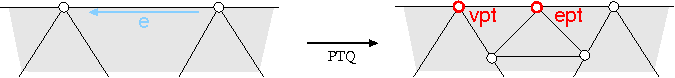CGAL 4.12 - 3D Surface Subdivision Methods
CGAL::Loop_mask_3< PolygonMesh, VertexPointMap > Class Template Reference

#include <CGAL/Subdivision_method_3/subdivision_masks_3.h>

## Definition

### template<class PolygonMesh, class VertexPointMap = typename boost::property_map<PolygonMesh, vertex_point_t>::type> class CGAL::Loop_mask_3< PolygonMesh, VertexPointMap >

The geometry mask of Loop subdivision.

A stencil determines a source neighborhood whose points contribute to the position of a refined point. The geometry mask of a stencil specifies the computation on the nodes of the stencil. Loop_mask_3 implements the geometry masks of Loop subdivision on a triangulated model of MutableFaceGraph, such as Polyhedron_3 and Surface_mesh.

Template Parameters
 PolygonMesh must be a model of the concept MutableFaceGraph. Additionally all faces must be triangles. VertexPointMap must be a model of WritablePropertyMap with value type Point_3Is Model Of:
PTQMask_3
CGAL::Subdivision_method_3

## Public Types

typedef PolygonMesh Mesh

typedef Halfedge_around_face_circulator< Mesh > Halfedge_around_facet_circulator

typedef Halfedge_around_target_circulator< Mesh > Halfedge_around_vertex_circulatorPublic Types inherited from CGAL::PQQ_stencil_3< PolygonMesh, VertexPointMap >
typedef PolygonMesh Mesh

typedef boost::property_map< Mesh, vertex_point_t >::type Vertex_pmap

typedef boost::graph_traits< Mesh >::vertex_descriptor vertex_descriptor

typedef boost::graph_traits< Mesh >::halfedge_descriptor halfedge_descriptor

typedef boost::graph_traits< Mesh >::face_descriptor face_descriptor

typedef boost::property_traits< Vertex_pmap >::value_type Point

typedef Kernel_traits< Point >::Kernel Kernel

typedef Kernel::FT FT

typedef Kernel::Vector_3 Vector

## Creation

Constructor. More...

Constructor with a custom vertex point property map.

## Stencil functions

void edge_node (halfedge_descriptor edge, Point &pt)
computes the Loop edge-point pt of the edge edge.

void vertex_node (vertex_descriptor vertex, Point &pt)
computes the Loop vertex-point pt of the vertex vertex.

void border_node (halfedge_descriptor edge, Point &ept, Point &vpt)
computes the Loop edge-point ept and the Loop vertex-point vpt of the border edge edge.Public Member Functions inherited from CGAL::PQQ_stencil_3< PolygonMesh, VertexPointMap >
PQQ_stencil_3 (Mesh *pmesh)

PQQ_stencil_3 (Mesh *pmesh, VertexPointMap vpmap)

void face_node (face_descriptor, Point &)

void edge_node (halfedge_descriptor, Point &)

void vertex_node (vertex_descriptor, Point &)

void border_node (halfedge_descriptor, Point &, Point &)Public Attributes inherited from CGAL::PQQ_stencil_3< PolygonMesh, VertexPointMap >
Mesh * pmesh

VertexPointMap vpmap

## Constructor & Destructor Documentation

The default vertex point property map, get(vertex_point, pmesh), is used.